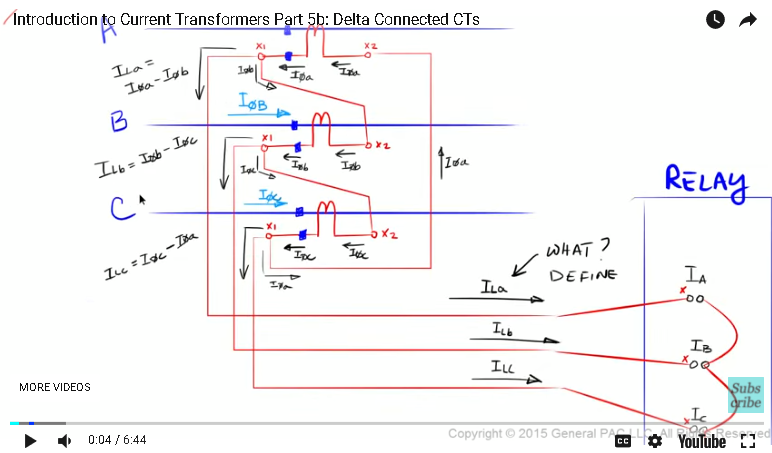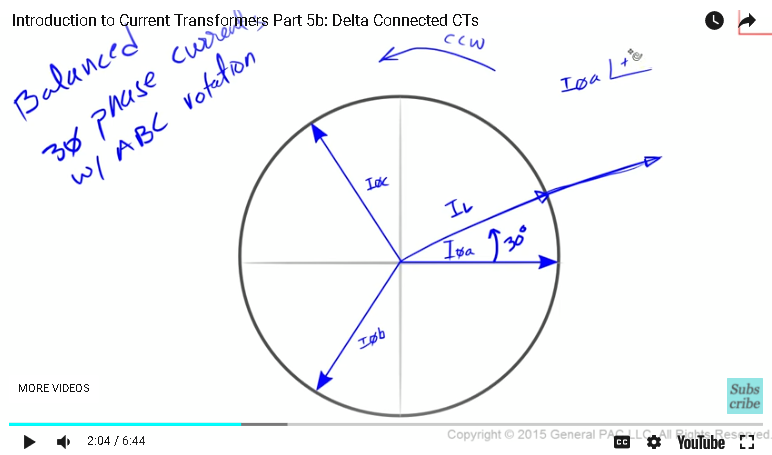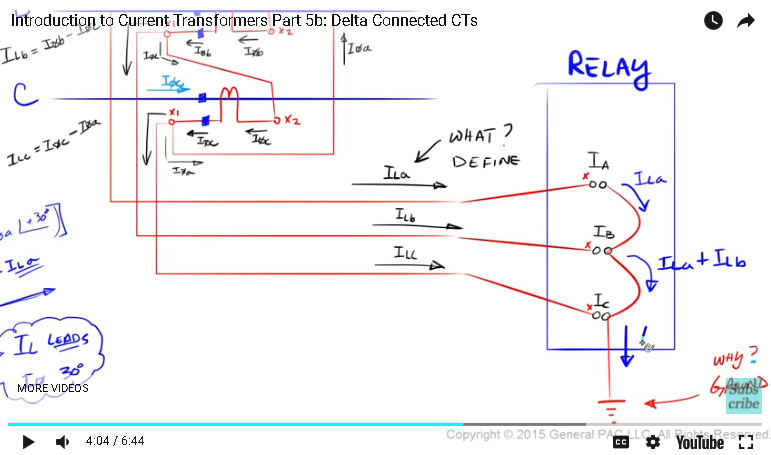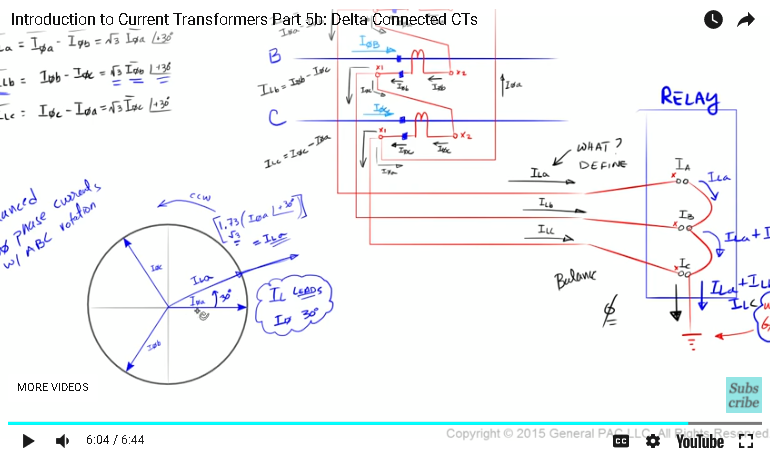This video was brought to you by GeneralPAC.com, making power systems Intuitive, Open and Free for Everyone, Everywhere. Consider subscribing and supporting through patreon.com/GeneralPAC. This is a mechanism for you to support us financially so we can continue making high quality power system video tutorials. Our corporate sponsor for this topic is AllumiaX.com from Seattle, Washington. Contact them for industrial and commercial power system studies.

Introduction to current transformers part five b

Now let's take a couple of minutes to actually look at these values from a phasor diagram perspective. So suppose we have this circle and then we have the x axis and the y axis drawn like this. And suppose that we were drawing a balanced set of three phase currents with A, B, C phase rotation, which means we'll define this as phase A, this as phase B, and this as phase C. So the equations that we're going to evaluate is essentially ILA is equal to I phase A minus I phase B and ILB is equal to I phase B minus I phase C and ILC is equal to I phase C minus I phase A.Okay. So let's evaluate the first one. ILA is essentially equal to the square root of three times I phase A rotated by 30 degrees plus 30 degrees in the counterclockwise direction. So these are all vectors. Similarly, line current B is equal to the square root of three times the phase current B rotated in the plus 20 degrees counterclockwise direction. And ILC, or the line current C, is equal to the square root of three times the phase C current rotated 30 degrees in the counterclockwise direction.

So from a phasor diagram's perspective, where all of the currents were rotating in the counterclockwise direction, line current A essentially starts at the origin and goes out like that. And to get line current A, we would have to take phase current A, we would have to rotate phase current A by 30 degrees, which puts phase current A at this mark here and then we would have to stretch phase current A by 173% to make it overlap line current A. So again, what we're essentially doing is we're taking phase current A, we are shifting phase current A by 30 degrees in the counterclockwise direction, and then we're stretching phase current A by 173% or 1.73, which is essentially the square root of three. And once we do that, we get the line current A. Pretty straight forward, right? With just a little bit of practice, this will become second nature.All right. So a little bit of observations. All three phase currents are rotating in the counterclockwise direction, which means that line current leads the phase current by 30 degrees. It's important to note this characteristic about this particular delta connection. The line current leads the phase current by 30 degrees.

Similar to what we did with line current A, we do the same exact procedure with line current B and line current C, which is this right here. So with line current B, we essentially take phase current B, we rotate it by 30 degrees in the counterclockwise direction, and we multiply phase current B by the square root of three to get line current B. And we do the same thing with line current C.

All right. So the last part of this video is essentially what happens to this line current. Now that we've defined this line current, what happens to this line current when the non-polarity side of our input terminal are connected like this? Okay. So we know that line current A is going into the polarity side of the input terminal for phase A, which means line current A is also flowing out of the non-polarity side of the input terminal. Similarly, the current that's flowing out of the non-polarity side of our phase B input terminal is line current A plus line current B, which makes sense, right? Line current B is flowing into the polarity side here. Line current A is flowing into the non-polarity side there. And what's flowing out is the sum of what's flowing in.

And lastly, what's flowing to ground is line current A plus line current B plus line current C. Let's move it over here. Okay. What if I told you that the current that's actually flowing to ground, this current here, what if I told you that current was zero? Under any sort of condition. What if I told you the current that's flowing to ground is zero meaning that there's no current flowing to ground at all? Would you believe me? Here's why I think under any sort of condition whether it's balanced or unbalanced, the current that's flowing to ground here is essentially zero. Well, let's look at it here. So we know that ILA plus ILB plus ILC, well ILA essentially equally I phase A minus I phase B. And then ILB equals I phase B minus I phase C. And ILC equals I phase C minus I phase A. What I'm saying is that this current is equal to zero. It's equal to zero because I phase A here and then negative I phase A is there. So that cancels out. I phase B is here and negative I phase B is there. So this cancels out. And I phase C is here and the negative I phase C is there. So this cancels out.So under any sort of condition, we don't have any current that's actually flowing to ground. So the question comes back to why do we ground this at all? This will be covered in a different module. So in this module, we drew three CTs and then connected them in delta. We labeled all of our phase currents. We labeled our line current. We defined our line currents. And we showed that our line current is a lot larger than our phase current. And to be exact, it's the square root of three times larger under balanced conditions. And the line current leads the phase current by 30 degrees if everything was rotating in the counterclockwise direction. So the line current leads the phase current by 30 degrees.And then of course we wrote down our relationship between line current and phase current. In part six, we're going to look at the CT model and how it looks from the theoretical perspective. Thank you. This module is brought to you by GeneralPac.com. Making power system protection, automation, and controls intuitive.

#### Greetings from the GeneralPAC Team!

We make high-quality Power Systems Video Tutorials on complex topics that are free and open to everyone!  Thank you so much for supporting us through Patreon so we can continue doing good and valuable work.

What is Patreon and why do we use it?

Patreon is a fantastic portal that allows our fans and community to make monthly contribution (like Netflix subscription) so we can continue creating high-quality power systems video tutorials. In return, you get access to incredible perks like voting on future topics, getting your questions answered, access to VIP Q/A webinars with the creators of GeneralPAC, and much more! We THANK YOU for supporting us

Why do we need your support?

An incredible amount of time and effort is needed to develop high-quality video tutorials. Each video (Part 1 for example) takes approximately 10 hours to complete which includes learning the concept ourselves, brainstorming creative ways to teach and explain the concepts, writing the script, audio recording, video recording, and editing. It's no wonder why Hundreds-of-Thousands of people have watched, liked, subscribed, and left positive comments on Youtube channel. Your support truly makes all the difference.